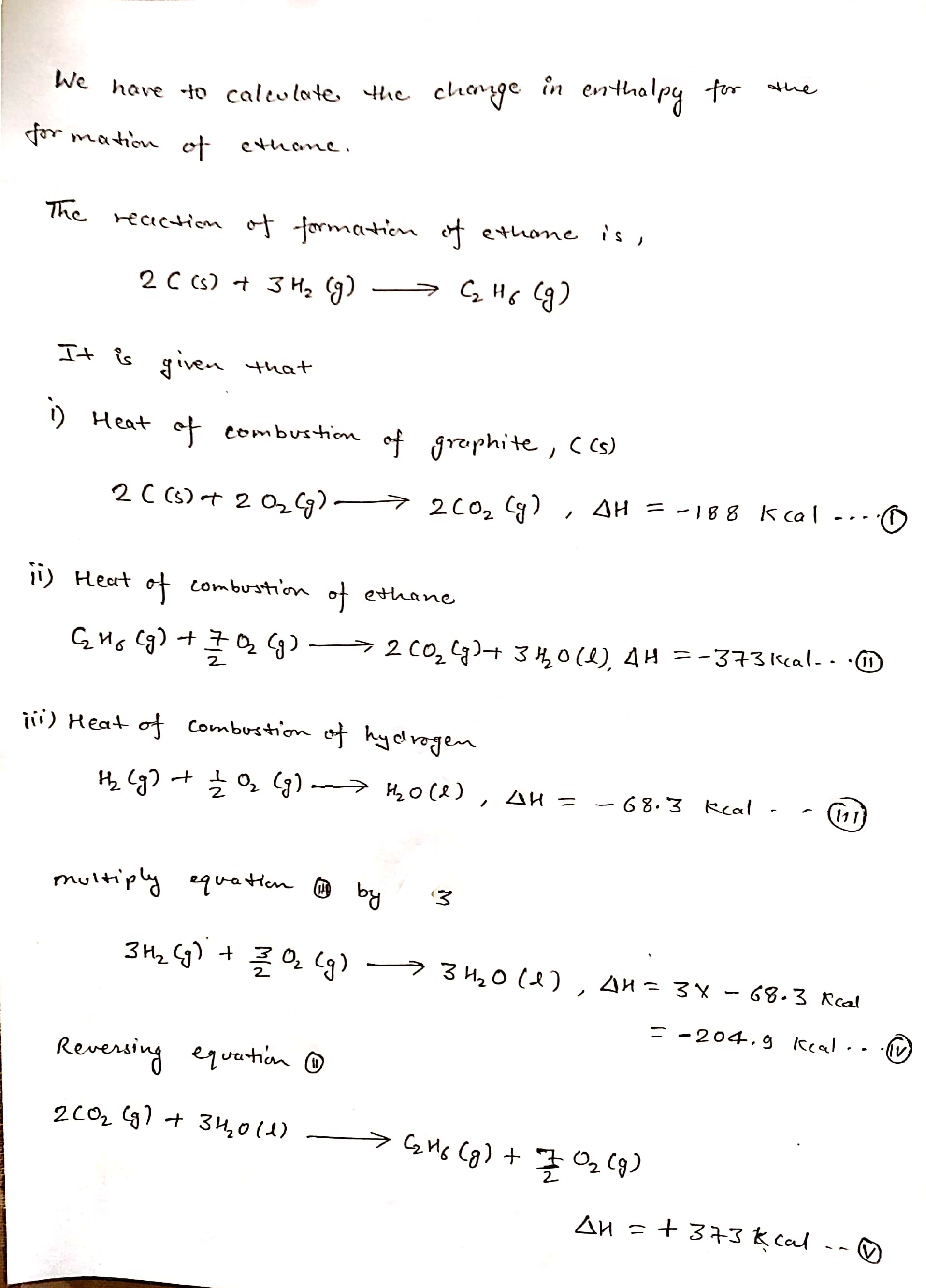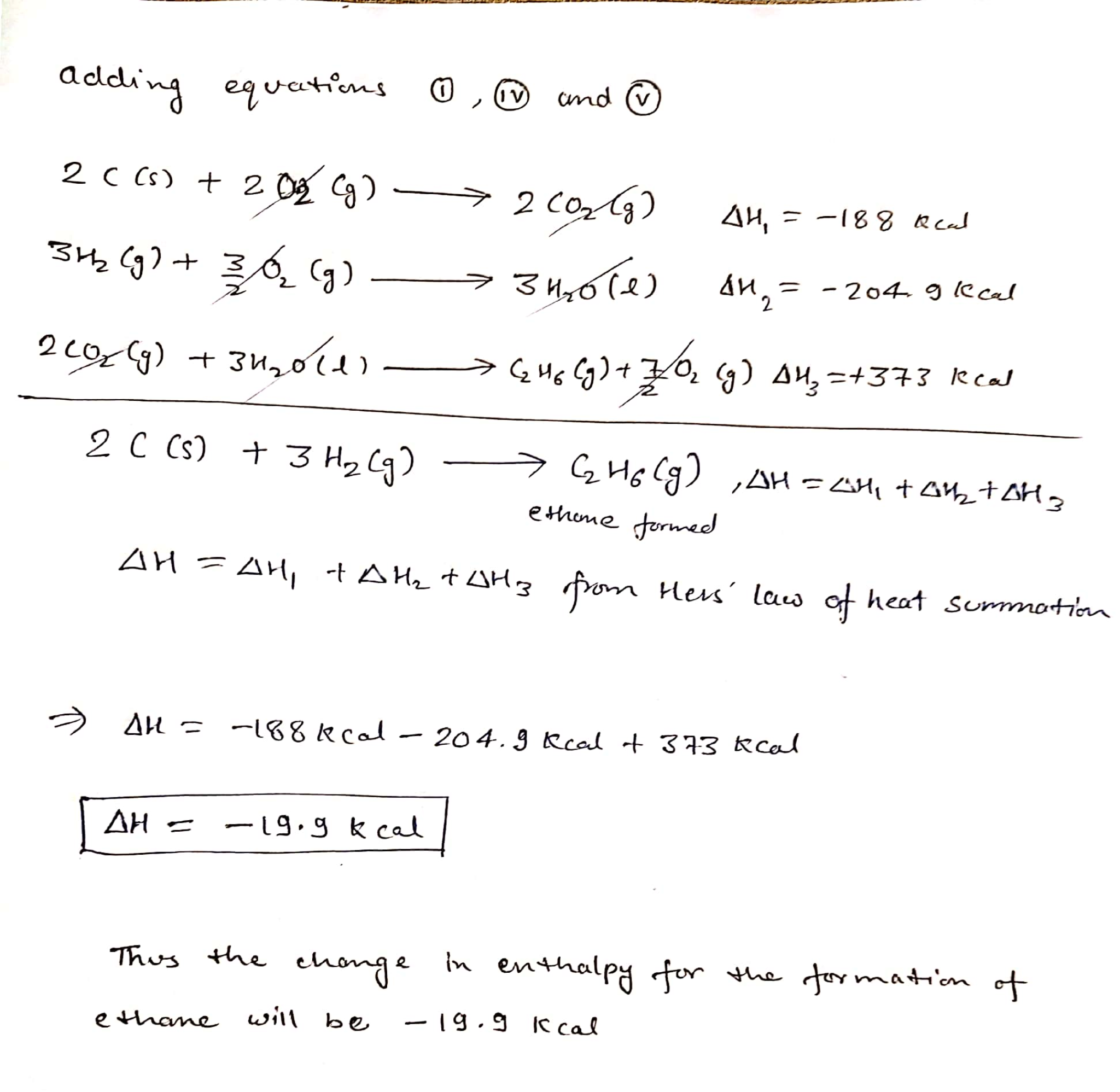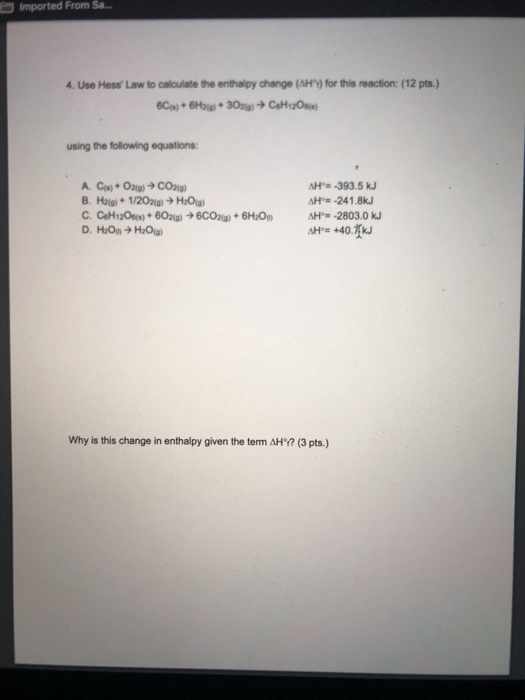# Calculate the change in enthalpy (ΔH) for the heat of formation of ethane, C2H6, using Hess' Law and the following reactions:

Calculate the change in enthalpy (ΔH) for the heat of formation of ethane, C2H6, using Hess' Law and the following reactions:

a) 2C(S) + 2O2(g) → 2CO2(g), ΔH = -188 kcal

b) C2H6(g) + (7/2)O2(g) → 2CO2(g) + 3H2O(l), ΔH = -373 kcal

c) H2(g) + (1/2)O2(g) → H2O(l), ΔH = -68.3 kcalAccording to the Hess' Law of constant heat summation, if we wish to calculate the heat of formation of a product which is formed in multiple steps then the required change in the enthalpy will be sum of change in enthalpies of every individual step.

Thankyou

##### Add Answer to: Calculate the change in enthalpy (ΔH) for the heat of formation of ethane, C2H6, using Hess' Law and the following reactions:
Similar Homework Help Questions
• ### Calculate the standard enthalpy change for producing ethane (C2H6) from its elements:

2C(s) + 3H2(g) –––––––> C2H6(g) ?H° = ???(In practice this reaction would be difficult to run as there would be many products)We know the standard enthalpy changes for the following combustion reactions::a) C2H6(g) + 7/2O2(g) –––––> 2CO2(g) + 3H2O(l) ?H° = – 1,559.7kJb) C(s) + O2(g) ––––––> CO2(g) ?H° = – 393.5kJc) H2(g) + 1/2O2(g) ––––––> H2O(l) ?H° = – 285.8kJPlease help me figure this out. Thank you.

• ### problem is together. 3. Calculate the enthalpy of formation of carbon dioxide in the following reaction:...problem is together. 3. Calculate the enthalpy of formation of carbon dioxide in the following reaction: C(s) + O2(g) - CO, (g) Use the following equations: a) H2O(l) → H2(g) + 2O2(g) AH°-= +285.8 kJ/mol b) C2H6(g) → 2C (s) + 3H2(g) AH'= +84.7 kJ/mol c) 2CO2 (g) +3H2O (1) C2H6(g) + (7/2) O2(g) AH°F = +1560.7 kJ/mol

• ### Use the enthalpies for the given reactions to compute the standard enthalpy change (ΔH°) for the...

Use the enthalpies for the given reactions to compute the standard enthalpy change (ΔH°) for the reaction: 6 C (s) + 7 H2(g) → C6H14 (l) ΔHrxn = ? C6H14 (l)+19/2O2 (g)→6CO2(g)+ 7H2O(g). ΔH=–3505.8kJ C(s) + O2 (g) → CO2(g)   ΔH=–393.5kJ H2(g) + 1/2 O2(g) → H2O(g).   ΔH = – 242.0 kJ

• ### 4. Use Hess' Law to calculate the enthalpy change (∆H°f) for this reaction: 6C(s) + 6H2(g)...

4. Use Hess' Law to calculate the enthalpy change (∆H°f) for this reaction: 6C(s) + 6H2(g) + 3O2(g)  C6H12O6(s) using the following equations: A. C(s) + O2(g)  CO2(g) ∆H°= -393.5 kJ B. H2(g) + 1/2O2(g)  H2O(g) ∆H°= -241.8kJ C. C6H12O6(s) + 6O2(g)  6CO2(g) + 6H2O(l) ∆H°= -2803.0 kJ D. H2O(l)  H2O(g) ∆H°= +40.7 kJ Why is this change in enthalpy given the term ∆H°f?

• ### 1- Why is this change in enthalpy given the term ∆H°f? 2-  Use Hess' Law to calculate...

1- Why is this change in enthalpy given the term ∆H°f? 2-  Use Hess' Law to calculate the enthalpy change (∆H°f) for this reaction: 6C(s) + 6H2(g) + 3O2(g)  C6H12O6(s) using the following equations: A. C(s) + O2(g) _____CO2(g) ∆H°= -393.5 kJ B. H2(g) + 1/2O2(g) ______H2O(g) ∆H°= -241.8kJ C. C6H12O6(s) + 6O2(g) _____ 6CO2(g) + 6H2O(l) ∆H°= -2803.0 kJ D. H2O(l) ________H2O(g) ∆H°= +40.7 kJ

• ### Use the enthalpies for the given reactions to compute the standard enthalpy change (ΔH°) for the...

Use the enthalpies for the given reactions to compute the standard enthalpy change (ΔH°) for the reaction C4H6 (g) + 2 H2 (g) → C4H10 (g) C4H6 (g) + 11/2 O2 (g) → 4 CO2 (g) + 3 H2O (l) ΔH° = −1937 kJ H2 (g) + 1/2 O2 (g) → H2O (l) ΔH° = −285.8 kJ C4H10 (g) + 13/2 O2 (g) → 4 CO2 (g) + 5 H2O (l) ΔH° = −2219.1 kJ

• ### change rH for reaction

Calculate delta or change rH for the reaction 2C(s) + 3H2(g) ---------- C2H6(g)given the following: As part of your answer, show how the equations can be added together to give the overall or net equationC2H6(g) + 7/2O2(g) --- 2CO2(g) + 3H2O(l) delta symbol or change rH = -1559.7 kJC(s) + O2(g) -------------CO2(g) delta or change rH = -393.5 kJH2(g) 1/2O2(g) ----------------H2O(l) delta or change rH = -285.8 kJ

• ### 4. Use Hess' Law to calculate the enthalpy change (Hºt) for this reaction: (12 pts.) 6C(s)...4. Use Hess' Law to calculate the enthalpy change (Hºt) for this reaction: (12 pts.) 6C(s) + 6H2(g) + 302(g) → CeH12O6(s) using the following equations: A. C(s) + O2(g) → CO2(g) B. H2(g) + 1/2O2(g) → H2O(g) C. CH12O6(s) + 602(g) → 6CO2(g) + 6H2O D. H2O → H2O() AH° -393.5 kJ AH°= -241.8kJ AH = -2803.0 kJ AH°= +40.7 kJ Why is this change in enthalpy given the term AH°? (3 pts.) Show transcribed image text

• ### 4. Use Hess' Law to calculate the enthalpy change (AHI) for this reaction: (12 pts.) 6C(s)...4. Use Hess' Law to calculate the enthalpy change (AHI) for this reaction: (12 pts.) 6C(s) + 6H2(g) + 302(g) → C6H12O6(s) using the following equations: A. C(s) + O2(g) → CO2(g) B. H2(g) + 1/2O2(g) → H2O(g) C. C6H12O6(s) + 6O2(g) → 6CO2(g) + 6H2O(1) D. H2O() → H2O(g) AH'= -393.5 kJ AH'= -241.8kJ AH°= -2803.0 kJ Hº= +40.7 kJ Why is this change in enthalpy given the term AHür? (3 pts.) Show transcribed image text

• ### Imported From Sa... 4. Use Hess' Law to calculate the enthalpy change (AHY) for this reaction:...Imported From Sa... 4. Use Hess' Law to calculate the enthalpy change (AHY) for this reaction: (12 pts.) 6C) + 6H21 309) CH120) using the following equations: A. Cw+ O2(g) → CO2(0) B. Hag) + 1/2O2(g) → H2O(g) C. CoH12O6 + 602(0)+ 6CO2(g) + 6H2O D. H2O → H2O(g) AH'= -393.5 kJ SH-241.8kJ AH = -2803.0 kJ AH = +40.71 kJ Why is this change in enthalpy given the term AHY? (3 pts.)

Free Homework App